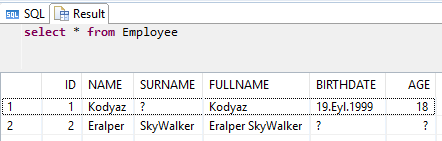﻿ Create Calculated Column in SAP HANA Database TablesSAP ABAP Programming and HANA Database Tutorials Development resources, articles, tutorials, code samples, tools and downloads for SAP HANA and ABAP, HANA Database, SQLScript, SAP UI5, Screen Personas, Web Dynpro, Workflow

# Create Calculated Column in SAP HANA Database Tables

"What is Calculated Column in Database Table and how to create calculated column in SAP HANA", in this HANA database tutorial I will try to answer these questions for ABAP developers. Calculated columns or computed columns are frequently used in other database platforms like SQL Server. Now SAP programmers working on SAP S/4HANA can work more closely with database features like calculated columns with HANA database and code push-down paradigm.

## What is Calculated Column?

Calculated columns in a SAP HANA database table can be considered as fields whose values are expressed as an output of a SQL expression which can use other column values and some other data as input parameters. Based on input parameters used in the calculated column expression the value of the computed field is calculated when the field is queried and read.

For example, assume that you are an ABAP programmer and store employee data in SAP HANA database table including birthdate of the employee.
It is not wise to store age in a persistent column in the HANA table because with passing time the age of the employee will increase.
So to make this calculation dynamic and return every time a valid result, HANA database developer can build a database model with calculated field for the employee table.

## Create Calculated Column on HANA Database Table

Let's create sample database table with calculated field "Age" using the BirthDate column value and current date function value as inputs to the SQLScript expression for the computed column

SQLScript syntax for creation of a HANA database table with calculated column is very similar to basic CREATE COLUMN TABLE syntax.
Only for the calculated field or computed column, SQL developers only define the column name then provide the SQL expression for the calculation right after "as"

For age calculation which I use for the Age calculated column, I used YEARS_BETWEEN() function and provided BirthDate column as first input parameter and today's date as the output of Current_Date HANA datetime function as the second input parameter.

Let's see how we can insert new rows and display calculated column in SAP HANA database table.

In the first data row provided with first INSERT command, I provided BirthDate column value as a valid date. But I did not insert a value for the Age column. But in the SELECT query results we will see Age is calculated and is being displayed.

In the second Insert statement, I did not provide a value for BirthDate. So it is not possible to calculate an age logically. To prevent errors at runtime it is better to think such cases during development and maake modifications during early phases of your development if necessary.
Luckily, SQLScript Years_Between datetime function returns null if any of the input parameters is NULL. So we actually handle correctly such cases.

Here is the output of the SELECT query executed on HANA table## More Calculated Column Samples in HANA Tables

Let's create additional calculated column in HANA using our sample database table Employee.
Remember we have Name field for FirstName and Surname field in our HANA table.
SQLScript developers frequently concatenate firstname and lastname for fullname during their developments especially for front-end applications.
Let's create a calculated column and set the computed field SQL expression once and afterwards ABAP developers can directly read fullname data from calculated table column easily without any SQL conversion, etc.

Here is the new CREATE TABLE command including modifications for the additional calculated column FullName in Emplyee sample HANA database table

Let's populate our sample database table with some test data and then query to see how computed column values are displayed using basic SQL Select statement.

Here is the data fetched using SQL Select queryFor concatenation of name parts, HANA SQL developers can see from above SQLScript I used CONCAT string function and IFNULL SQL function together to provide a space character between name parts for all possible combinations like the case that surname is not provided since the table column is nullable.

## Restrictions Related with Calculated Column Creation

Although using calculated columns can be attractive for most cases for SQL developers, there are many restrictions preventing SQLScript programmers to use different computed column expressions.

For example, HANA database developers can not use user defined functions in calculated column expression.

Here is a user-defined function that I created to read salary value for a given Employee Id

Although I can use the custom SQL function without any error on my SQLScript commands as follows;

But using the same user-defined function in following CREATE TABLE command will trigger SQL exception

Here is the error:

SAP DBTech JDBC: : unsupported function included: unsupported function type for generated column: user defined function

A similar problem is with subquery usage in calculated column expression. If you try to use a subquery expression, you will experience following SQL exception

SAP DBTech JDBC: : feature not supported: cannot support this type of expression: subquery in calculated field

SQL Server provides more flexibility to database developers on Computed Columns like using UDF (User Defined Functions) and enabling calculated field value to be stored just like other table columns using PERSISTED clause.

SAP HANA and ABAP